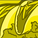# QlikView App Dev

Discussion Board for collaboration related to QlikView App Development.

cancel
Showing results for
Did you mean:Creator III

## Set Analisys in expression for more values

Hi i have in my chart expression, this set analisys:

Sum({1<YEAR={'\$(=getfieldselections(YEAR))'},SELLER={'\$(=getfieldselections(SELLER))'}, DES_REFER={'\$(=getfieldselections(DES_REFER))'} >} BUD_VOLUMI)

if i choose one Year one seller and one des_refer, all is ok and work good, if i choose more des_refer, or more years it not work

what the problem in the expression?

2 Solutions

Accepted SolutionsMVP

Try this

``Sum({1<YEAR = p(YEAR), SELLER = p(SELLER), DES_REFER =p(DES_REFER)>} BUD_VOLUMI)``Creator III
Author

no sorry in this way it not work, but i find like write it good with concat:

Sum({1<YEAR={\$(=chr(39) & concat(distinct YEAR, chr(39) &', ' & chr(39)) & chr(39) )}>} BUD_VOLUMI)

7 RepliesContributor III

Maybe is for this (in red color, copied from Qlik help):

GetFieldSelections - chart function

GetFieldSelections() returns a string with the current selections in a field.

If all but two, or all but one of the values are selected, the format 'NOT x,y' or 'NOT y' will be used respectively. If you select all values and the count of all values is greater than max_values, the text ALL will be returned.

Try using concat instead.

Hope it helps.MVP

Try this

``Sum({1<YEAR = p(YEAR), SELLER = p(SELLER), DES_REFER =p(DES_REFER)>} BUD_VOLUMI)``Creator III
Author
Thanks, but why in my set analisys not work? .... i use getfieldselections as right, if i use it in a text box i see it write all values i chooseCreator III
Author
Can you write an example with concat function using my set analisys, for understand what you mean? so i can try it if workContributor III

I mean this:

Sum({1<YEAR={'\$(=concat(YEAR,','))'},SELLER={'\$(=concat(SELLER,','))'}, DES_REFER={'\$(=concat(DES_REFER,','))'} >} BUD_VOLUMI)

Concat returns a string with all the values of the field, separated by the char you say in the second parameterCreator III
Author

no sorry in this way it not work, but i find like write it good with concat:

Sum({1<YEAR={\$(=chr(39) & concat(distinct YEAR, chr(39) &', ' & chr(39)) & chr(39) )}>} BUD_VOLUMI)Contributor III

Yes. You are right. This is the point when using special chars like ' in \$ expansion expressions.

If my idea was useful, please check my suggestion as a solution.